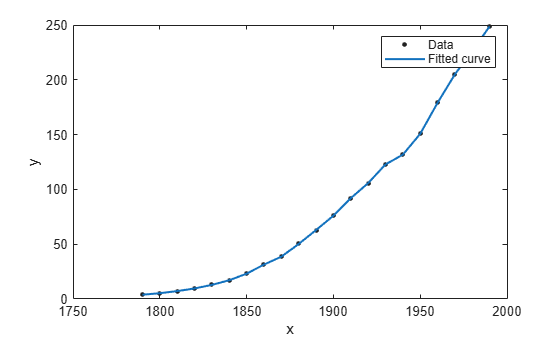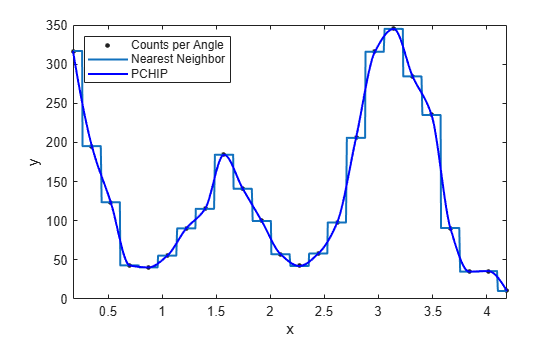## Selecting an Interpolant Fit

### Select Interpolant Fit Interactively

Open the Curve Fitter app by entering `curveFitter` at the MATLAB® command line. Alternatively, on the Apps tab, in the Math, Statistics and Optimization group, click Curve Fitter.

On the Curve Fitter tab, in the Fit Type section, select an Interpolant fit. When you select an Interpolant fit, the app fits an interpolating curve or surface that passes through every data point.

In the Fit Options pane, you can specify the Method value.For curve data, you can set Method to `Linear`, `Nearest neighbor`, ```Cubic Spline```, or `Shape-preserving (PCHIP)`. For surface data, you can set Method to ```Nearest neighbor```, `Linear`, `Cubic Spline`, `Biharmonic (v4)`, or ```Thin-plate spline```.

For surfaces, the Interpolant fit uses the `scatteredInterpolant` function for the `Linear` and `Nearest neighbor` methods, the `griddata` function for the `Cubic Spline` and `Biharmonic (v4)` methods, and the `tpaps` function for the `Thin-plate spline` method. Try the ```Thin-plate spline``` method when you require both smooth surface interpolation and good extrapolation properties. For more details, see About Interpolation Methods.

Tip

If your data variables have very different scales, select and clear the Center and scale check box to see the difference in the fit. Normalizing the inputs can strongly influence the results of the triangle-based (that is, piecewise `Linear` and `Cubic Spline` interpolation) and ```Nearest neighbor``` surface interpolation methods.

### Fit Linear Interpolant Models Using the `fit` Function

This example shows how to use the `fit` function to fit linear interpolant models to data.

Interpolant Fitting Methods

Specify the interpolant model method when calling the fit function using one of the options outlined in Interpolant Model Names. None of the interpolant methods has any additional fit option parameters.

Fit a Linear Interpolant Model

Load data and fit a linear interpolant model using the `'linearinterp'` option.

```load census f = fit(cdate,pop,'linearinterp'); plot(f,cdate,pop);```Compare Linear Interpolant Models

Load data and create both nearest neighbor and pchip interpolant fits using the `'nearestinterp'` and `'pchip'` options.

```load carbon12alpha f1 = fit(angle,counts,'nearestinterp'); f2 = fit(angle,counts,'pchip');```

Compare the fitted curves `f1` and `f2` on a plot.

```p1 = plot(f1,angle,counts); xlim([min(angle),max(angle)]) hold on p2 = plot(f2,'b'); hold off legend([p1;p2],'Counts per Angle','Nearest Neighbor','pchip',... 'Location','northwest')```For an alternative to 'cubicinterp' or 'pchipinterp', you can use other spline functions that give you greater control over what you create. See Introducing Spline Fitting.